# The Lyapunov exponent

A quantitative measure of the sensitive dependence on the initial conditions is the Lyapunov exponent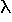. It is the averaged rate of divergence (or convergence) of two neighboring trajectories. Actually there is a whole spectrum of Lyapunov exponents. Their number is equal to the dimension of the phase space. If one speaks about the Lyapunov exponent, the largest one is meant. It is important because it determines the prediction horizon. Even qualitative predictions are impossible for a time interval beyond this horizon. It is given by ln(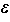)/max, whereis the error of the measurment of the initial state. Here, the error is given in units of the averaged amplitude of the nonperiodic oscillation.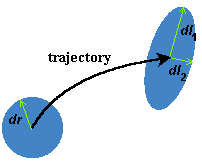To obtain the Lyapunov spectra, imagine an infinitesimal small ball with radius dr sitting on the initial state of a trajectory. The flow will deform this ball into an ellipsoid. That is, after a finite time t all orbits which have started in that ball will be in the ellipsoid. The ith Lyapunov exponent is defined by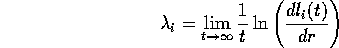where dli(t) is the radius of the ellipsoid along its ith principal axis.

 QUESTIONS worth to think about: Lyapunov exponents can be defined also for other non-wandering sets like fixed points etc. How can we calculate the Lyapunov exponent for fixed points? For the driven pendula, one Lyapunov exponent is always zero and at least one is always negative. Why?

© 1998 Franz-Josef Elmer,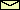Franz-Josef doht Elmer aht unibas doht ch, last modified Friday, July 24, 1998.